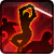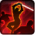# Master StrikeConditions

Used by

## Related effects, buffs and debuffs

• [hidden] [does not expire]Effect #1

 Slot: Debuff Duration: passive Tick rate: does not tick # occurrences: 0 Tags: tag.​abl.​jedi_knight.​master_strike, tag.​abl.​jedi_knight.​melee_ability
• When effect ticks the 1th time
• When effect ticks the 2th time

Only when the following conditions are met:

• If difficulty is any of 8-man HM, 16-man SM

Perform the following actions:

• Weapon Damage
- Is Special Ability = (bool) true
- Ignore Dual Wield Modifier = (bool) true
- Flurry Blows Min = (int) 1
- Flurry Blows Max = (int) 1
- Level Cap = (int) 0
- Threat Percent = (float) 0
- Standard Health Percent Max = (float) 0.08
- Standard Health Percent Min = (float) 0.07
- Amount Modifier Fixed Max = (float) 0
- Amount Modifier Fixed Min = (float) 0
- Amount Modifier Percent = (float) -1
- Coefficient = (float) 0
- Health Steal Percentage = (float) 0
- Slots = Int(5)
• When effect ticks the 1th time
• When effect ticks the 2th time

Only when the following conditions are met:

• If difficulty is any of 8-man SM, 8-man HM, 16-man SM, 16-man HM

Perform the following actions:

• Weapon Damage
- Is Special Ability = (bool) true
- Ignore Dual Wield Modifier = (bool) true
- Flurry Blows Min = (int) 1
- Flurry Blows Max = (int) 1
- Level Cap = (int) 0
- Threat Percent = (float) 0
- Standard Health Percent Max = (float) 0.04
- Standard Health Percent Min = (float) 0.03
- Amount Modifier Fixed Max = (float) 0
- Amount Modifier Fixed Min = (float) 0
- Amount Modifier Percent = (float) -1
- Coefficient = (float) 0
- Health Steal Percentage = (float) 0
- Slots = Int(5)
• When effect ticks the 3th time

Only when the following conditions are met:

• If difficulty is any of 8-man SM, 8-man HM, 16-man SM, 16-man HM

Perform the following actions:

• Weapon Damage
- Is Special Ability = (bool) true
- Ignore Dual Wield Modifier = (bool) true
- Flurry Blows Min = (int) 1
- Flurry Blows Max = (int) 1
- Level Cap = (int) 0
- Threat Percent = (float) 0
- Standard Health Percent Max = (float) 0.24
- Standard Health Percent Min = (float) 0.2
- Amount Modifier Fixed Max = (float) 0
- Amount Modifier Fixed Min = (float) 0
- Amount Modifier Percent = (float) 0
- Coefficient = (float) 1
- Health Steal Percentage = (float) 0
- Slots = Int(5)
• When effect ticks the 3th time

Only when the following conditions are met:

• If difficulty is any of 8-man HM, 16-man SM

Perform the following actions:

• Weapon Damage
- Is Special Ability = (bool) true
- Ignore Dual Wield Modifier = (bool) true
- Flurry Blows Min = (int) 1
- Flurry Blows Max = (int) 1
- Level Cap = (int) 0
- Threat Percent = (float) 0
- Standard Health Percent Max = (float) 0.24
- Standard Health Percent Min = (float) 0.2
- Amount Modifier Fixed Max = (float) 0
- Amount Modifier Fixed Min = (float) 0
- Amount Modifier Percent = (float) -1
- Coefficient = (float) 1
- Health Steal Percentage = (float) 0
- Slots = Int(5)
• Just before this effect is removed
• [target override]

Perform the following actions:

• Play appearance epp . jedi_knight . master_strike . cancel